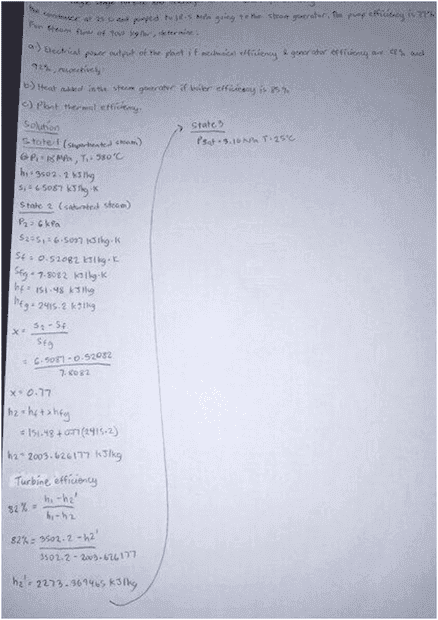# Rankine cycle power plant

• Engineering
Kamuna
Homework Statement:
determine:
a) Electrical power output of the plant if mechanical efficiency & generator efficiency
are 88 % & 92 %, respectively.
b) Heat added in the steam generator if boiler efficiency is 85 %
c) Plant thermal efficiency
Relevant Equations:
Rankine cycle
Is this the right path in solving this problem? Am i finding the correct enthalpies? I am also having difficulty understanding so what I've understood is that in a rankine cycle P2=P3 but here P2 is given and P3 has a given variable which is T=25 C too so should I neglect P2=P3?

Heres my problem set and the attempt to solve it:

In a Rankine cycle power plant superheated steam enters the turbine at 18 MPa and 580 C. Steam expands through a single stage turbine and discharges at 6 kPa with stage efficiency of 82 %. The condensate leaves the condenser at 25 C and pumped to 18.5 MPa going to the steam generator. The pump efficiency is 77 %. For steam flow of 9,000 kg/hr, determine:

• Electrical power output of the plant if mechanical efficiency & generator efficiency
• are 88 % & 92 %, respectively.
• Heat added in the steam generator if boiler efficiency is 85 %
• Plant thermal efficiency
Any help would be much appreciated, thanks#### Attachments

Last edited by a moderator: